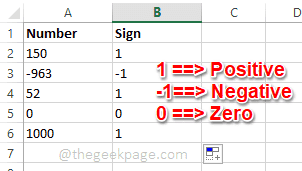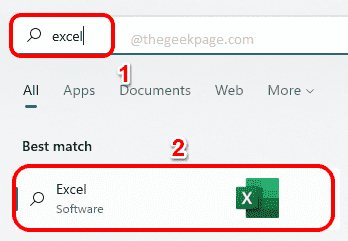# How to Use SIGN Function to Find Out Whether a Number is Positive or Negative in Excel

The SIGN function is an extremely useful function that comes inbuilt with Microsoft Excel. Using this function, you can find out the sign of a number. i.e., whether the number is positive or not. The SIGN function returns 1 if the number is positive, returns -1 if the number is negative and returns zero if the number is zero. Though it may sound too obvious, if there is a huge column with many rows containing many numbers and we want to find the signs of them all, it’s really useful to use the SIGN function and get the job done in a matter of seconds.

In this article, we explain through 3 different methods how you can easily use the SIGN function in any excel document to figure out the signs of numbers. Read on, to learn how you can master this cool trick.## Launch Microsoft Excel

To try out any of the methods listed in this article, firstly you need to launch Microsoft Excel. For that, click on the Search icon on the Taskbar.Then type in excel in the Search bar and then click on Excel application from the list of applications in the search results.Now, let’s see how we can find the sign of a number in Excel.

## Method 1: By Typing in Formula

Step 1: Once Excel launches open, click on the cell where you want to see the sign of the number. Now type in =SIGN(<column_row_id>) and hit Enter key.

Please replace <column_row_id> with the column and row combination id of the cell containing the number that you want to find the sign of.

For example, in the screenshot below, I have written my formula as =SIGN(A2) because A2 is the column and row combination id of the cell that contains the number 150 that I want to find the sign of.Step 2: Hitting Enter in the previous step would give you the sign of the number you want, in the cell that you selected.

Now, if you want to expand the formula to other cell values as well, you just have to click on the solid square dot at the corner of the cell containing the SIGN formula and drag it down.Step 3: Viola! Now you have the signs of all the numbers in the table just as simple as that.

If the SIGN function gives 1, it means that the number is Positive.

If the SIGN function gives -1, it means that the number is Negative.

If the SIGN function gives 0, it means the number Zero and it doesn’t have a sign.## Method 2: By Using Insert Function in Formulas Tab

Step 1: Firstly, click on the cell where you want the result to come.

As next, click on the FORMULAS tab in the top ribbon and then click on the Insert Function button.Step 2: As next, from the Insert Function window, under Select a function section, click on the function named SIGN. Here you will get to see the definition of the SIGN function.

```SIGN(number)

Returns the sign of a number: 1 if the number is positive, zero if the number is zero, or -1 if the number is negative.```

Note: The (number) part of the SIGN function is the argument that needs to be passed to this function. It is the sign of this argument that the SIGN function finds out.

Once you are all done, hit the OK button.Step 3: Once you click the OK button, in the Number field you can enter the id of the cell that contains the number, of which you want to find the sign.

Hit the OK button once you have entered the cell id.Step 4: Once you have the result of one cell, you can click on the square dot at the corner of the cell to span the function to other cells as well.Step 5: That’s it! Now you have the sign results for all the numbers that you require.## Method 3: By Using Math & Trig Dropdown in Formulas Tab

Step 1: Firstly, click on the cell where you want the result to be processed.

Click on the FORMULAS tab at the top and then under it, click on the Math & Trig dropdown menu.Step 2: As next, from the dropdown menu, scroll down, find and click on the function named SIGN.Step 3: In the Function Arguments window, under the Number field, type in the cell id where your number is present, that you want to find the sign for. Hit the OK button after entering the cell id.Step 4: Once you have the SIGN result in your selected cell, just like in the previous methods, you can drag and span the function across all the cells under it.Step 5: There you go! You have your results all ready right before you! Enjoy!That’s it. Hope you found the article useful.